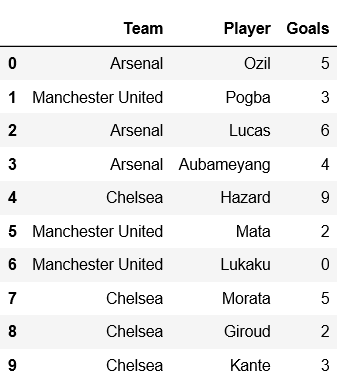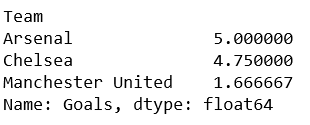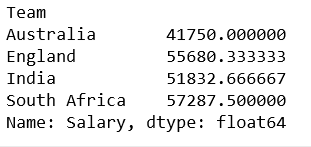# Grouping Rows in pandas

• Difficulty Level : Basic
• Last Updated : 14 Jan, 2019

Pandas is the most popular Python library that is used for data analysis. It provides highly optimized performance with back-end source code is purely written in C or Python.

Let’s see how to group rows in Pandas Dataframe with help of multiple examples.

Example 1:

For grouping rows in Pandas, we will start with creating a pandas dataframe first.

 `# importing Pandas``import` `pandas as pd`` ` `# example dataframe``example ``=` `{``'Team'``:[``'Arsenal'``, ``'Manchester United'``, ``'Arsenal'``,``                   ``'Arsenal'``, ``'Chelsea'``, ``'Manchester United'``,``                   ``'Manchester United'``, ``'Chelsea'``, ``'Chelsea'``, ``'Chelsea'``],``                    ` `           ``'Player'``:[``'Ozil'``, ``'Pogba'``, ``'Lucas'``, ``'Aubameyang'``,``                       ``'Hazard'``, ``'Mata'``, ``'Lukaku'``, ``'Morata'``, ``                                         ``'Giroud'``, ``'Kante'``],``                                          ` `           ``'Goals'``:[``5``, ``3``, ``6``, ``4``, ``9``, ``2``, ``0``, ``5``, ``2``, ``3``] }`` ` `df ``=` `pd.DataFrame(example)`` ` `print``(df)`Now, create a grouping object, means an object that represents that particular grouping.

 `total_goals ``=` `df[``'Goals'``].groupby(df[``'Team'``])`` ` `# printing the means value``print``(total_goals.mean())    `

Output:Example 2:

 `import` `pandas as pd`` ` `# example dataframe``example ``=` `{``'Team'``:[``'Australia'``, ``'England'``, ``'South Africa'``,``                   ``'Australia'``, ``'England'``, ``'India'``, ``'India'``,``                        ``'South Africa'``, ``'England'``, ``'India'``],``                         ` `           ``'Player'``:[``'Ricky Ponting'``, ``'Joe Root'``, ``'Hashim Amla'``,``                     ``'David Warner'``, ``'Jos Buttler'``, ``'Virat Kohli'``,``                     ``'Rohit Sharma'``, ``'David Miller'``, ``'Eoin Morgan'``,``                                                 ``'Dinesh Karthik'``],``                                                  ` `          ``'Runs'``:[``345``, ``336``, ``689``, ``490``, ``989``, ``672``, ``560``, ``455``, ``342``, ``376``],``           ` `          ``'Salary'``:[``34500``, ``33600``, ``68900``, ``49000``, ``98899``,``                    ``67562``, ``56760``, ``45675``, ``34542``, ``31176``] }`` ` `df ``=` `pd.DataFrame(example)`` ` `total_salary ``=` `df[``'Salary'``].groupby(df[``'Team'``])`` ` `# printing the means value``print``(total_salary.mean())     `

Output:My Personal Notes arrow_drop_up